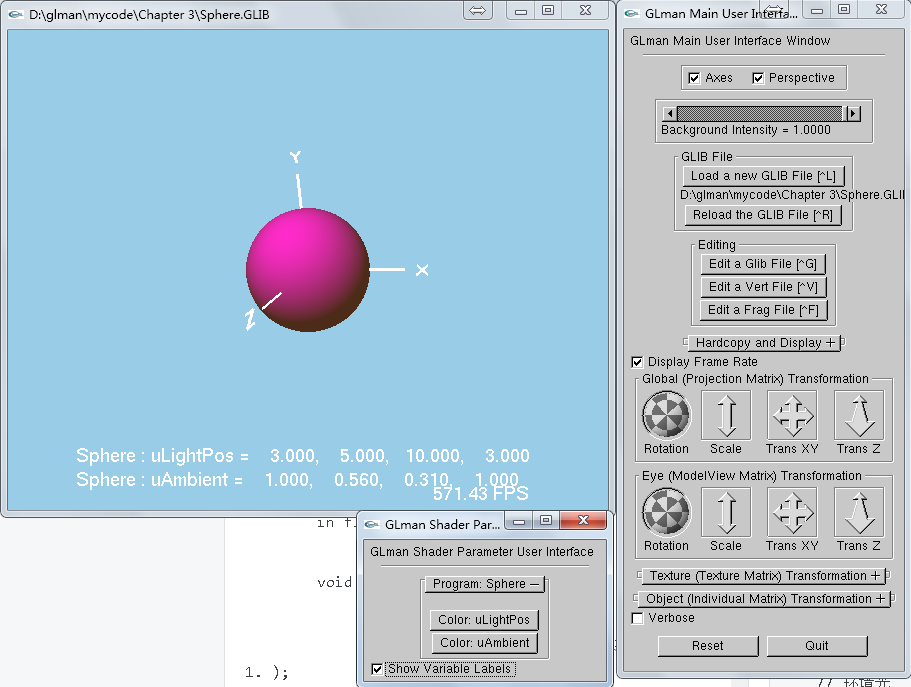• 书名：图形着色器——理论与实践(第2版)
• 原名：Graphics Shaders: Theory and Practice
• 作者：Mike Bailey, Steve Cunnungham
• 翻译：刘鹏
• 出版社：清华大学出版社
• ISBN：978-7-302-31599-5

### 学习笔记

glman 使用GLIB来描述一个着色器程序。`GLIB` 文件与jME3的 `j3md` 文件很相似，都可以用来定义uniform变量。不过 GLIB

### 第一个着色器程序

`Sphere.GLIB` 文件：

``````WindowSize 600 480
LookAt 0 0 4  0 0 0  0 1 0
Perspective 90 // 透视相机
Background 0.6 0.8 0.9 1.0 // 背景色

Vertex Sphere.vert
Fragment Sphere.frag
Program Sphere \
uLightPos {3. 5. 10.} \
uAmbient {1. 0.56 0.31 1.}

Color 1. 0. 1.
Sphere 1. 100 100
``````

``````uniform vec4 uLightPos;

out vec4 vColor;
out vec3 vMCposition;
out float vLightIntensity;

void main() {
vec3 transNormal = normalize( gl_NormalMatrix * gl_Normal.xyz);
vec3 ECposition = vec3( gl_ModelViewMatrix * gl_Vertex );
vec3 ECLightPosition = vec3 ( gl_ModelViewMatrix * uLightPos );
vLightIntensity = dot( normalize( ECLightPosition - ECposition ), transNormal );
vLightIntensity = clamp( vLightIntensity, 0., 1. );
vColor = gl_Color;
vMCposition = vec3( gl_Vertex );
gl_Position = vec4( gl_ModelViewProjectionMatrix * gl_Vertex );
}
``````

``````uniform vec4 uAmbient;

in vec4 vColor;
in float vLightIntensity;

void main() {
// 环境光
vec4 ambient = vec4( uAmbient.rgb, 1. );
// 漫反射
vec4 diffuse = vec4( vColor.rgb * vLightIntensity, 1.);
// 合成光
gl_FragColor = ambient * 0.3 + diffuse * 0.7;
}
``````### 第二个着色器程序

`QuadXY.GLIB` 文件

``````WindowSize 600 480
LookAt 0 0 4  0 0 0  0 1 0
Perspective 90 // 透视相机

uHeight <0.01 0.15 0.3> \
uScale <1 5 8>

Color 1.0 0.8 0.0
``````

``````uniform float uScale;
uniform float uHeight;

out float vLightIntensity;
out vec4 vColor;

const vec3 LIGHTPOS = vec3(0., 10., 0.);

void main() {
vec4 thisPos = gl_Vertex;
vColor = gl_Color;

// create a new height for this vertex
float lengthSquared = dot(thisPos.xy, thisPos.xy) * uScale;

// the Surface is z= uHeight * sin(x^2 + y^2)
thisPos.z = uHeight * sin( lengthSquared );

vec3 xtangent = vec3( 1.0, 0.0, 0.0);
xtangent.z = 2.0 * uHeight * thisPos.x * cos( lengthSquared );

vec3 ytangent = vec3( 0.0, 1.0, 0.0);
ytangent.z = 2.0 * uHeight * thisPos.y * cos( lengthSquared );

vec3 thisNormal = normalize( cross( xtangent, ytangent ) );
vec3 ECposition = vec3( gl_ModelViewMatrix * gl_Vertex );

vLightIntensity = dot( normalize( LIGHTPOS - ECposition ), thisNormal );
vLightIntensity = 0.3 + abs( vLightIntensity );// 0.3 ambient
vLightIntensity = clamp( vLightIntensity, 0., 1. );

gl_Position = gl_ModelViewProjectionMatrix * thisPos;
}
``````

``````in vec4 vColor;
in float vLightIntensity;

void main() {
gl_FragColor = vec4( vColor.rgb * vLightIntensity, 1.);
}
``````